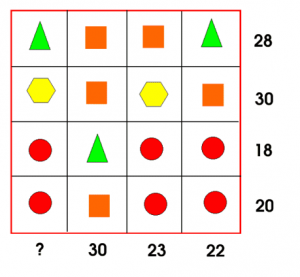# Tuesday 24th March – Maths – Year 6

Look at this shape puzzle:Each shape represents a number. Can you work out what number each shape represents and what the total is for the missing column?

### 16 thoughts on “Tuesday 24th March – Maths – Year 6”

•24th March 2020 at 12:07 pm

Triangle=7
Square=7
Hexagon=8
Circle=4
Total for missing colloum is 18

•24th March 2020 at 1:40 pm

Remember the rules of algebra – different shapes must be worth different totals.

•24th March 2020 at 12:51 pm

Square – 8

Triangle- 6

Circle – 4

Hexagon- 7

Missing total is 21

•24th March 2020 at 1:27 pm

Square is 8
Hexagon is 7
circle is 4 and triangle is 6 I think the missing total is 21

•24th March 2020 at 3:40 pm

Triangle=6
Square=8
Hexagon=7
Circle=4
I think that the missing column is 21.

•24th March 2020 at 3:45 pm

Circle=4
Triangle=6
Square=8
Hexagon=9
The ?question mark is 22

•24th March 2020 at 4:01 pm

Did my answer come through ?

•24th March 2020 at 6:27 pm

Circle=4
Square=8
Triangle=6
Hexagon=7
?=21

•25th March 2020 at 9:37 am

Triangle=6
Square =8
Circle =4
Hexagon =9
?=23

•25th March 2020 at 11:56 am

Triangle=6
Circle=4
Square=8
Hexagon=9
23

•25th March 2020 at 11:01 am

yellow = 7 green = 6 red = 12 orange =8 the answer in the bar is 37

•25th March 2020 at 11:04 am

red =4

•25th March 2020 at 11:05 am

•25th March 2020 at 11:38 am

triangle =6
square=8
circle=4
hexagon=7
missing number is 21

•25th March 2020 at 1:38 pm

circle=4
square=8
triangle=6
hexagon=7
and the missing number is 21

•